New update is available. Click here to update.
Last Updated: Jun 30, 2023
Easy

# Deque() in Python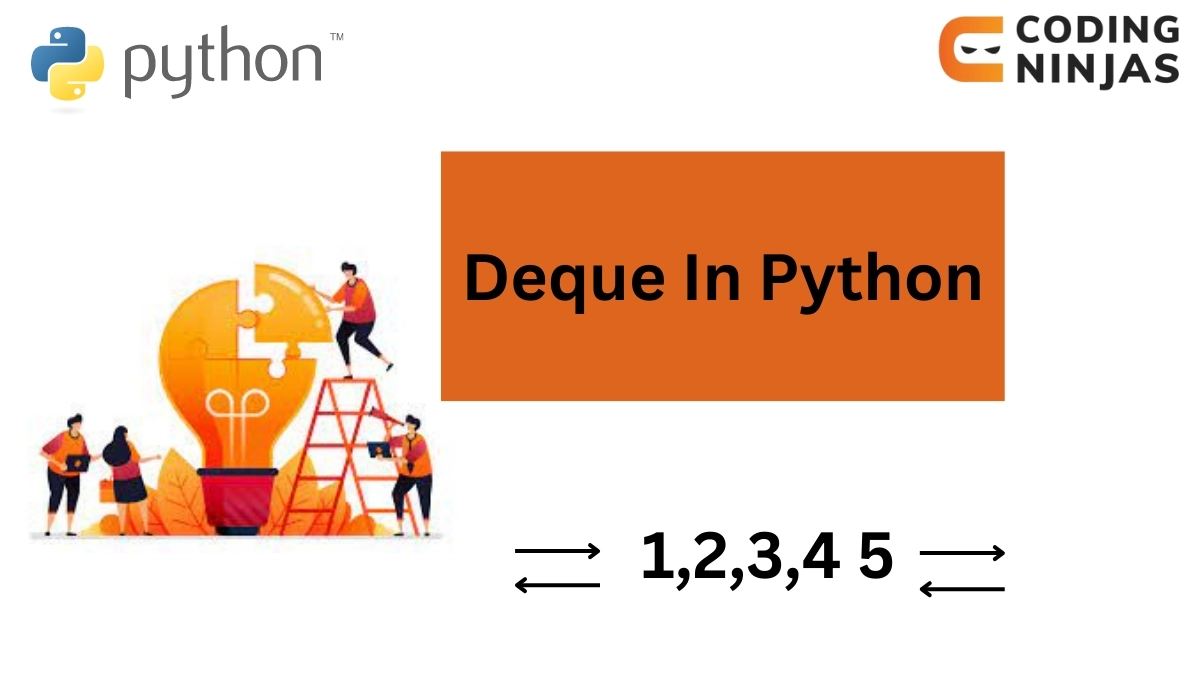## Introduction

Data Structures form the fundamental building blocks of various programs. Mastering Data Structures and Algorithms can open many doors for careers in big tech companies. In this article, we will discuss an important Data Structure called a Deque.

We are also going to the implementation of Deque() in Python. Before going into the specifics of Deque() in python, let us first look at what a Queue is.

Also see, Merge Sort Python and Convert String to List Python.

## Queue

A Queue is a linear data structure. It is very much like the Queues that we see in real life. It can be thought of as a list. All the additions in this list are made at only one end. Whereas all the removals are made from the other end.

A Queue is a FIFO(First in, First Out) data structure. A FIFO data structure is when the elements that are inserted first in the list are removed first. Like a regular queue, the person at the front gets serviced first.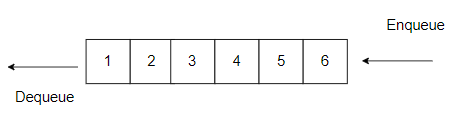There are two primary functions of a queue:

### Enqueue

Used to add new elements to the back of a queue.(Time complexity = O(1))

### Dequeue

Used to remove elements from the front of a queue.(Time Complexity = O(1))

Let us take a look at the implementation of Queue in Python:

## Queue In Python Using List

``````# Showing the functions of a queue using a list in python
queue = []

# Enqueue
queue.append(1)

# Enqueue
queue.append(2)

print("The Queue is:")
print(queue)

# Dequeue
print("The element popped from the queue is")
print(queue.pop(0))
print("Updated Queue")
print(queue)

'''
If the pop function is called on an empty queue, an error will be thrown
this is because there are no elements to access on the provided index
'''``````

Output: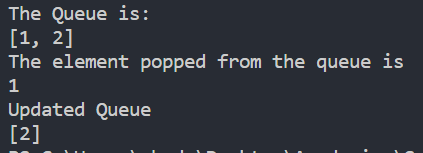Now, let us look at Deque and the implementation of deque in python.

You can practice by yourself with the help of online python compiler.

## What is Deque?

A Deque can be called a special case of Queue. It is also called a Double ended Queue. In a Deque data structure, elements can be added from both ends of the list. With that, the elements can also be removed from both ends.

Adding elements to a Deque() in Python is like adding elements to a list either at the beginning or end. Same with removing the elements from a deque. Both the addition and removal operations take constant time, making the time complexity O(1).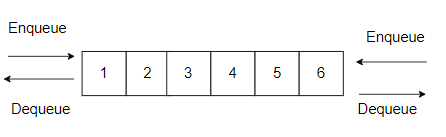Let us take a look at the implementation of deque in python.

Deque() in python can be implemented like a Queue in Python using a list.

Following is the implementation of Deque() in python using a list

Swapcase in Python

## Types of Restricted Deque Input

A deque is a data structure that allows you to insert and remove elements from both ends. But in a restricted deque input, there are some limitations on the type of operations that you can do.

There are two types of restricted deque input:

• Input-restricted deque: In this type of deque, you can only add elements to one end (the back end). But you can remove elements from both ends. It's like a queue where you can only add to the back of the line, but anyone can leave from the front or the back.
• Output-restricted deque: In this type of deque, you can only remove elements from one end (the front end). But you can add elements to both ends. It's like a stack where you can only take things off the top, but you can add things to the top or the bottom.

Both input and output-restricted deques have their own specific uses, depending on what you need to do with the data.

## Deque In Python Using List

``````# Showing the functions of a dequeue in python using a list
queue = []

# Enqueue at the right
queue.append(1)

# Enqueue at the right
queue.append(2)

# Enqueue at the left
queue.insert(0,3)

print("The Queue is:")
print(queue)

# Dequeue
print("The element popped from the queue is")

# Pop element from the left
print(queue.pop(0))
print("Updated Queue")
print(queue)

# Pop element from the right
print("The element popped from the queue is")
print(queue.pop(0))
print("Updated Queue")
print(queue)

'''
If the pop function is called on an empty queue, an error will be thrown
this is because there are no elements to access on the provided index
'''``````

Output: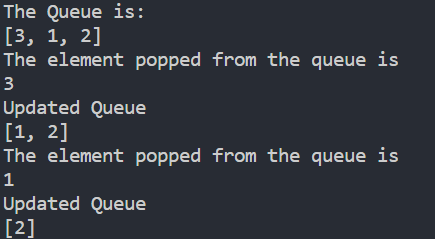Python also has an inbuilt module, which helps implement deque in python. Thus, it must be imported from the python collections to utilize that module. The module is called deque. The implementation of deque in python from collections can be done as follows:

## Deque In Python Using Collections

Let us look at some of the functions obtained for deque() in python from collections: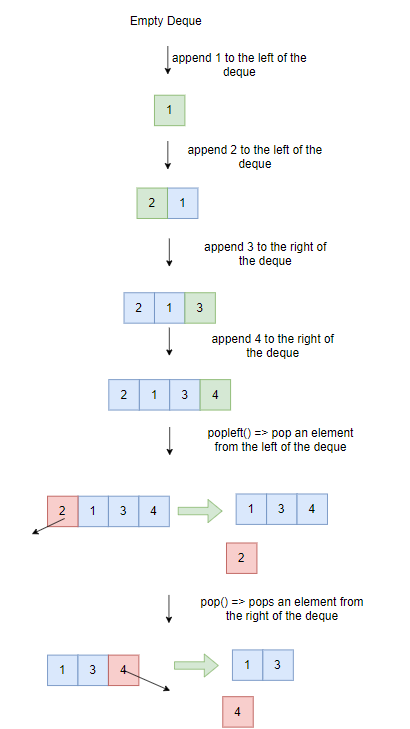Now let us take a look at it's implementation:

``````# Implementation of deque() in python from collections

from collections import deque

original_queue = []
n = int(input("Enter the Number of Elements in the Queue: "))
print("Enter the Numbers: ")

for i in range(n):
temp = int(input())
original_queue.append(temp)
dq = deque(original_queue)

# Insert element at the left of the deque
dq.appendleft(1)
dq.appendleft(2)

# Insert elements at the right of the deque
dq.append(3)
dq.append(4)

print("The data in the Deque is as follows:")
print(dq)

# Removing data from the left
print("Popped Element from the Left:")
print(dq.popleft())
print("Updated Deque")
print(dq)

# Removing data from the right
print("popped Element from the Right:")
print(dq.pop())
print("Updated Deque")
print(dq)``````

Output: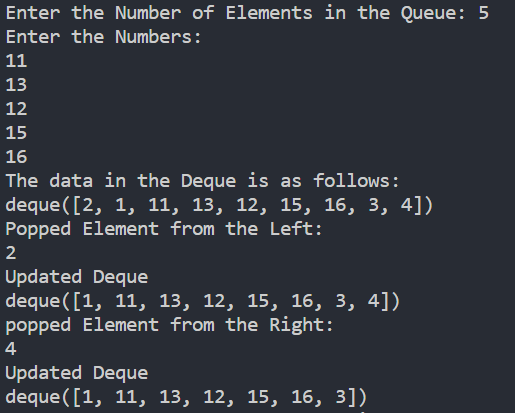Apart from the major functions of enqueuing and dequeuing elements, deque() in python offers some extra functionality as well, such as:

Let us take a look at the implementation of the above-mentioned functions:

## Additional Features For Deque In Python Using Collections

``````# Implementation of deque() in python from collections

from collections import deque

dq = deque([1,2,3,3,3,4,5,5,6,7])

print("The Deque is:")
print(dq)
print("\n")

# Insert element at the left of the deque
dq.appendleft(1)
dq.appendleft(2)

# Insert elements at the right of the deque
dq.append(3)
dq.append(4)

print("The data in the Deque is as follows:")
print(dq)
print("\n")

# Removing data from the left
print("Popped Element from the Left:")
print(dq.popleft())
print("Updated Deque")
print(dq)
print("\n")

# Removing data from the right
print("popped Element from the Right:")
print(dq.pop())
print("Updated Deque")
print(dq)
print("\n")

print("The first occurrence of element at 3 between the index 1 and 6 is at:")
print(dq.index(3,1,6))
print("\n")

print("Inserting 10 at index 2:")
dq.insert(2,10)
print("Updated Deque:")
print(dq)
print("\n")

print("Removing first occurrence of 5")
dq.remove(5)
print("Updated Deque:")
print(dq)
print("\n")

print("The Number of occurrences of the element 3 are: ")
print(dq.count(3))
print("\n")

print("Adding the list [0,-1,-2] to the right of the deque")
dq.extend([0,-1,-2])
print("Updated Deque")
print(dq)
print("\n")

print("Adding the list [10,11,12] to the left of the deque")
dq.extendleft([10,11,12])
print("Updated Deque")
print(dq)
print("\n")

print("Reversing the Deque")
dq.reverse()
print("Updated Deque")
print(dq)
print("\n")

print("Rotating the Deque to the left by 3")
dq.rotate(3)
print("Updated Deque")
print(dq)
print("\n")``````

Output: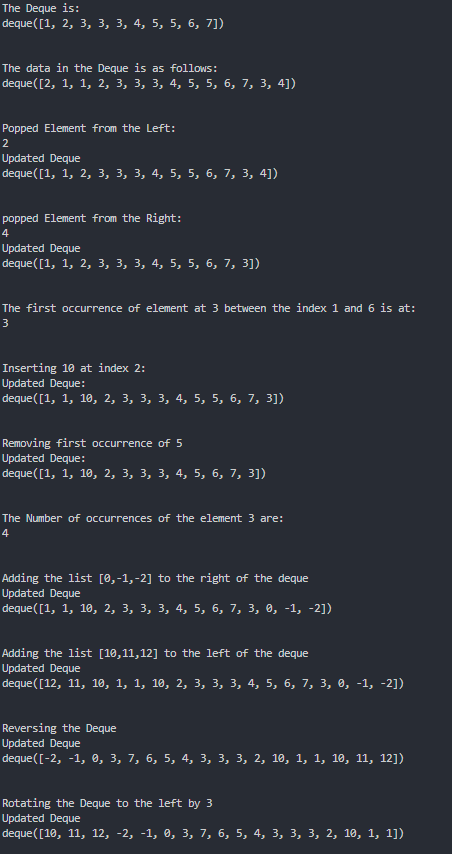## Building Efficient Queues With deque

We will understand this concept with a short example:

``````from collections import deque

queue = deque()
queue.append('apple')
queue.popleft()``````

This creates an empty queue using deque(), adds an element 'apple' to the queue using append(), and removes the first element from the queue using popleft(). The append() and popleft() methods have O(1) time complexity, making deque an efficient choice for implementing queues.

### How is deque implemented python?

Deque is implemented as a doubly-linked list in Python. Each element in the deque holds a reference to the preceding and following items, allowing for constant time operations to add or remove elements from both ends of the deque.

### Is Deque a linear data structure?

Yes, Deque, like Queue and Stack, is a linear data structure.

### What is the time complexity of inserting and removing an element from a deque at its ends?

An element can be added as well as removed at the ends of the deque in constant time. Thus the time complexity is O(1).

### How is a Stack different from a Deque?

Elements can be removed and added only to one end in a stack think of it as a stack of books. Whereas, in a Deque, elements can be added and removed at both ends.

## Conclusion

In this article, we briefly discussed what a Queue is. We also discussed Deque() in detail and the implementation of deque() in Python.